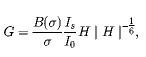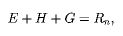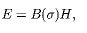# Solving 3 non linear algebraic equations in python

8244
35
11-28-2017 08:44 PMNew Contributor III

How can I solve a non-linear algebraic equation in ArcGIS python over multiple rasters. Let me Rephrase. I want to solve the following 3 non linear equations,andfor 46 8 day time steps.  I have 46 rasters each for an 8 day period for Β(σ) , and σ, where I need to take input values from per time step. Is/Io is a constant. The three unknowns I have are E, G and H. The solution should ideally produce 46 rasters each for the 3 unknowns. I don't know if this can be done in ArcGIS though. I know I can solve this equations easily in SciPy using the Fsolve function but I don't know how to get this working with rasters. Any code that could help will be greatly appreciated.

Tags (1)
35 RepliesbyMVP Legendary Contributor

If you can solve it with SciPy and the input takes 2D arrays then you can convert the raster to an array using

RasterToNumPyArray

the only concern is to ensure that the extent and cell sizes are the same.  If the data types are the same that is good, but the array can be promoted or demoted as necessary.

If the inputs require a 1D array, then you can 'ravel()' the 2D array.

Perhaps some more information would facilitate devising a workflow to permit the integrationNew Contributor III

Hi Dan,

While I thought it should be fairly easy with scipy...I was wrong. I wrote this basic part of the code however it didn't quite work with all the errors below and I am struggling to figure it out. As u can guess I am a newbie.

My struggles are now that I cant even get the fsolve to work. I found out scipy is included in ArcGIS 10.4.1 (which is my current version), so I imported it and tried my hands on it. As a sample instead of trying to work with rasters, I used single values for the raster component...with no success. The values 1.024 in F(0), 300 in F(1) and 3.356 in F(0) and  F(2) are daily rasters which I want the code to iterate through and pick the set for each day. There are 46 each in different folders.  I hope the information below can help explain the problems a bit more clearly. Thanks.

import scipy
import numpy as np
def myFunction(z):
...     E = z
...     G = z
...     H = z
...     F = empty((3))
...     F = (3.356/1.024)*3*H*pow((abs(H),-(1/6)))-G
...     F = 300-E-H-G
...     F = 3.356*H-E
...     return F

zGuess= np.array([1,1,1])
z = fsolve(myFunction,zGuess)
Traceback (most recent call last):
File "<ipython-input-29-eb6f86348f14>", line 1, in <module>
z = fsolve(myFunction,zGuess)
File "C:\Program Files\Anaconda3\lib\site-packages\scipy\optimize\minpack.py", line 146, in fsolve
res = _root_hybr(func, x0, args, jac=fprime, **options)
File "C:\Program Files\Anaconda3\lib\site-packages\scipy\optimize\minpack.py", line 212, in _root_hybr
shape, dtype = _check_func('fsolve', 'func', func, x0, args, n, (n,))
File "C:\Program Files\Anaconda3\lib\site-packages\scipy\optimize\minpack.py", line 26, in _check_func
res = atleast_1d(thefunc(*((x0[:numinputs],) + args)))
File "<ipython-input-27-7e512d399a9a>", line 5, in myFunction
F = empty((3))
NameError: name 'empty' is not definedbyMVP Legendary Contributor

immediate issues... I will deal with the rest after classes

empty... can't just doe that, it has to be np.empty

when you import numpy as np... as other functions should be preceeded by np... don't do an import all either to save two letterdNew Contributor III

Right! Makes sense. Noted. Thanks I'll wait for your response after your class.

CheersNew Contributor III

Hi Dan, I fixed the numpy thing as you said. I fiddled around with it a little and it appears to be working now, however, I still need help with coding it to take those rasters in sequentially and also to write each of the E, G and H out as individual raster for each time step it iterates through. See below the code that worked. Look forward to your response.

import arcpy
import scipy
import numpy as np
def myFunction(z):
...     E = z
...     G = z
...     H = z
...     F = np.empty((3))
...     F = (3.356/1.024)*3*H*pow(abs(H),-(1/6))-G
...     F = 300-E-H-G
...     F = 3.356*H-E
...     return F

zGuess= np.array([1,1,1])
z = fsolve(myFunction,zGuess)

print z
[  70.96121951  207.89419779   21.14458269]byMVP Legendary Contributor

just got back fsolve is in the scipy optimize minpack

notice how I imported fsolve on line 1.

I cut out some of the 'dir' information on line 4

The full help is on line 9 onwards.

So on first glance,it you have the 3 parameters then the tuple of 3 seems reasonable.  Interpreting it I suspect would be in the order that they were given.

If will look further soon, but this should keep you up to date

from scipy.optimize import fsolve

dir(fsolve)
Out: ['__annotations__', '__call__', '__class__...._name__', '__ne__', '__new__', '__qualname__', '__reduce__', '__reduce_ex__', '__repr__', '__setattr__', '__sizeof__', '__str__', '__subclasshook__']

help(fsolve)

Help on function fsolve in module scipy.optimize.minpack:

fsolve(func, x0, args=(), fprime=None, full_output=0, col_deriv=0, xtol=1.49012e-08, maxfev=0, band=None, epsfcn=None, factor=100, diag=None)
Find the roots of a function.

Return the roots of the (non-linear) equations defined by
func(x) = 0 given a starting estimate.

Parameters
----------
func : callable f(x, *args)
A function that takes at least one (possibly vector) argument.
x0 : ndarray
The starting estimate for the roots of func(x) = 0.
args : tuple, optional
Any extra arguments to func.
fprime : callable(x), optional
A function to compute the Jacobian of func with derivatives
across the rows. By default, the Jacobian will be estimated.
full_output : bool, optional
If True, return optional outputs.
col_deriv : bool, optional
Specify whether the Jacobian function computes derivatives down
the columns (faster, because there is no transpose operation).
xtol : float, optional
The calculation will terminate if the relative error between two
consecutive iterates is at most xtol.
maxfev : int, optional
The maximum number of calls to the function. If zero, then
100*(N+1) is the maximum where N is the number of elements
in x0.
band : tuple, optional
If set to a two-sequence containing the number of sub- and
super-diagonals within the band of the Jacobi matrix, the
Jacobi matrix is considered banded (only for fprime=None).
epsfcn : float, optional
A suitable step length for the forward-difference
approximation of the Jacobian (for fprime=None). If
epsfcn is less than the machine precision, it is assumed
that the relative errors in the functions are of the order of
the machine precision.
factor : float, optional
A parameter determining the initial step bound
(factor * || diag * x||).  Should be in the interval
(0.1, 100).
diag : sequence, optional
N positive entries that serve as a scale factors for the
variables.

Returns
-------
x : ndarray
The solution (or the result of the last iteration for
an unsuccessful call).
infodict : dict
A dictionary of optional outputs with the keys:

nfev
number of function calls
njev
number of Jacobian calls
fvec
function evaluated at the output
fjac
the orthogonal matrix, q, produced by the QR
factorization of the final approximate Jacobian
matrix, stored column wise
r
upper triangular matrix produced by QR factorization
of the same matrix
qtf
the vector (transpose(q) * fvec)

ier : int
An integer flag.  Set to 1 if a solution was found, otherwise refer
to mesg for more information.
mesg : str
If no solution is found, mesg details the cause of failure.

--------
root : Interface to root finding algorithms for multivariate
functions. See the 'hybr' method in particular.

Notes
-----
fsolve is a wrapper around MINPACK's hybrd and hybrj algorithms.

‍‍‍‍‍‍‍‍‍‍‍‍‍‍‍‍‍‍‍‍‍‍‍‍‍‍‍‍‍‍‍‍‍‍‍‍‍‍‍‍‍‍‍‍‍‍‍‍‍‍‍‍‍‍‍‍‍‍‍‍‍‍‍‍‍‍‍‍‍‍‍‍‍‍‍‍‍‍‍‍‍‍‍‍‍‍‍‍‍‍‍‍‍‍‍‍‍‍‍‍‍‍‍‍‍‍‍‍‍‍‍‍‍‍‍‍‍‍‍‍‍‍‍‍‍‍‍‍‍‍‍‍‍‍‍‍‍‍‍‍‍‍‍‍‍‍‍‍‍‍‍‍‍‍‍‍‍‍‍‍‍‍‍‍‍‍‍‍‍‍‍‍‍‍‍‍‍‍‍‍‍‍‍‍‍‍‍‍‍‍‍‍‍New Contributor III

Yes Dan thanks. I forgot to include the line that I used to import the fsolve function in scipy. I also agree that the results are written in the order of input. From here I guess it's how to iterate through through the folders to pick the inputs and also write out the outputs as rasters that will seal the work!New Contributor III

After getting the code to run using just numbers to replace the rasters, I tried this code but it didn't quite work. Please help. This is doing my head in. I tried this code which I basically tried to cut out a chunk of a code that once worked in iteration. Please help. I am running out of time for a dealine

import os

... import arcpy

... import scipy

... import numpy as np

... def myFunction(z):

... E = z

... G = z

... H = z

... F = np.empty((3))

... F = (file/fize)*3*H*pow(abs(H),-(1/6))-G

... F = fixe-E-H-G

... F = file*H-E

... return F

...

... zGuess= np.array([1,1,1])

... arcpy.env.workspace ="D:/GIS Data/Sixth Creek ET.gdb"

... rasters = arcpy.ListRasters("*", "All")

... path = "C:\\Workspace\\test geowrite\\Betasigma\\"

... path2 = "C:\\Workspace\\test geowrite\\sigma\\"

... files= [f for f in os.listdir(path) if f.endswith(".tif")]

... fizes= [f for f in os.listdir(path2) if f.endswith(".tif")]

... fixes= [f for f in os.listdir(path2) if f.endswith(".tif")]

... for i in range(0,46):

... file = path+files

... fize = path2+fizes

... fixe = path3+fixes

... z = fsolve(myFunction,zGuess)

...

Runtime error

Traceback (most recent call last):

File "<string>", line 28, in <module>

File "C:\Program Files (x86)\python27\ArcGIS10.4\lib\site-packages\scipy\optimize\minpack.py", line 140, in fsolve

res = _root_hybr(func, x0, args, jac=fprime, **options)

File "C:\Program Files (x86)\python27\ArcGIS10.4\lib\site-packages\scipy\optimize\minpack.py", line 197, in _root_hybr

shape, dtype = _check_func('fsolve', 'func', func, x0, args, n, (n,))

File "C:\Program Files (x86)\python27\ArcGIS10.4\lib\site-packages\scipy\optimize\minpack.py", line 20, in _check_func

res = atleast_1d(thefunc(*((x0[:numinputs],) + args)))

File "<string>", line 10, in myFunction

TypeError: unsupported operand type(s) for /: 'str' and 'str'New Contributor III

Xander Bakker,@Dan Patterson, Randy burton, @kevin Dunlop, @Joshua Bixby   After getting the code to run using just numbers to replace the rasters, I tried this code but it didn't quite work. Please help. This is doing my head in. I tried this code which I basically tried to cut out a chunk of a code that once worked in iteration. Please help. I am running out of time for a dealine

import os

... import arcpy

... import scipy

... import numpy as np

... def myFunction(z):

... E = z

... G = z

... H = z

... F = np.empty((3))

... F = (file/fize)*3*H*pow(abs(H),-(1/6))-G

... F = fixe-E-H-G

... F = file*H-E

... return F

...

... zGuess= np.array([1,1,1])

... arcpy.env.workspace ="D:/GIS Data/Sixth Creek ET.gdb"

... rasters = arcpy.ListRasters("*", "All")

... path = "C:\\Workspace\\test geowrite\\Betasigma\\"

... path2 = "C:\\Workspace\\test geowrite\\sigma\\"

... files= [f for f in os.listdir(path) if f.endswith(".tif")]

... fizes= [f for f in os.listdir(path2) if f.endswith(".tif")]

... fixes= [f for f in os.listdir(path2) if f.endswith(".tif")]

... for i in range(0,46):

... file = path+files

... fize = path2+fizes

... fixe = path3+fixes

... z = fsolve(myFunction,zGuess)

...

Runtime error

Traceback (most recent call last):

File "<string>", line 28, in <module>

File "C:\Program Files (x86)\python27\ArcGIS10.4\lib\site-packages\scipy\optimize\minpack.py", line 140, in fsolve

res = _root_hybr(func, x0, args, jac=fprime, **options)

File "C:\Program Files (x86)\python27\ArcGIS10.4\lib\site-packages\scipy\optimize\minpack.py", line 197, in _root_hybr

shape, dtype = _check_func('fsolve', 'func', func, x0, args, n, (n,))

File "C:\Program Files (x86)\python27\ArcGIS10.4\lib\site-packages\scipy\optimize\minpack.py", line 20, in _check_func

res = atleast_1d(thefunc(*((x0[:numinputs],) + args)))

File "<string>", line 10, in myFunction

TypeError: unsupported operand type(s) for /: 'str' and 'str'